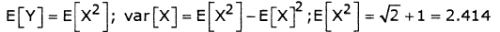Courses

# Digital Communications - MCQ Test - 1

## 15 Questions MCQ Test GATE ECE (Electronics) 2022 Mock Test Series | Digital Communications - MCQ Test - 1

Description
This mock test of Digital Communications - MCQ Test - 1 for Electronics and Communication Engineering (ECE) helps you for every Electronics and Communication Engineering (ECE) entrance exam. This contains 15 Multiple Choice Questions for Electronics and Communication Engineering (ECE) Digital Communications - MCQ Test - 1 (mcq) to study with solutions a complete question bank. The solved questions answers in this Digital Communications - MCQ Test - 1 quiz give you a good mix of easy questions and tough questions. Electronics and Communication Engineering (ECE) students definitely take this Digital Communications - MCQ Test - 1 exercise for a better result in the exam. You can find other Digital Communications - MCQ Test - 1 extra questions, long questions & short questions for Electronics and Communication Engineering (ECE) on EduRev as well by searching above.
QUESTION: 1

###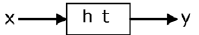has P.S.D. Sxx f then y has P.S.D

Solution: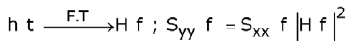QUESTION: 2

### Consider Y= ax+b, a>0. If the density and distribution functions of x are F. (x) and fx (x), then Fy (y) is equal to

Solution: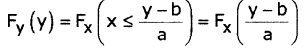QUESTION: 3

### Consider a random process x(t). Which of the following can represent Rx. (r)?

Solution: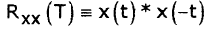*Answer can only contain numeric values
QUESTION: 4

It is required to transmit at 100 Kbit/s and a bandwidth of 2 MHz is used. The

minimum S/N ratio is ___________________

Solution: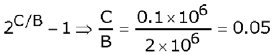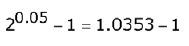*Answer can only contain numeric values
QUESTION: 5

SNR when the system is uncoded is 4 dB, when it is coded is 2 dB then the coding gain is ________________  (dB)

Solution:

Coding gain =  SNR encoded - SNR. coded = 4.dB-2dB = 2dB

QUESTION: 6

In the set up shown below, determine the number of bits required to encode each sample of x (t) such that bandwidth of signal y(t) is greater than 5 MHz.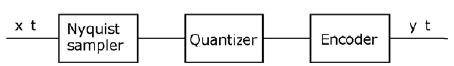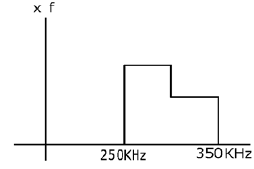Solution:

Nyquist sampler is a sampler that samples a given signal at a rate twice the highest frequencies

fs = 2 fm, f5 = 2 x 350 kHz = 0.7MHz

Band width of output of PCM =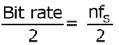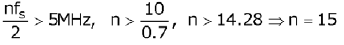QUESTION: 7

A sinusoidal signal whose peak-peak amplitude is 6 V is modulated using deltamodulator. At what rate should be sinusoidal signal sampled such that slope over load distortion is prevented with step size of 0.942 V?

Solution:

2A = 6V ⇒ A = 3V

In order to overcome slope over load distortion in delta modulator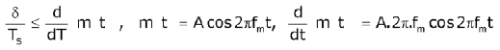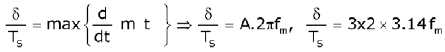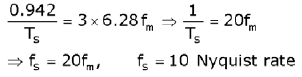QUESTION: 8

The probability of the five possible outcomes of an experiment are given as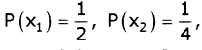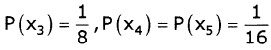Determine the informationrate, if there are 8 outcomes per second.

Solution: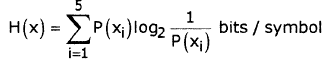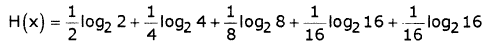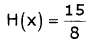= 1.875 bits / sec; r=8 outcomes/sec
Information rate R=r.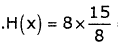=15bits / sec

QUESTION: 9

Probability of picking 3 symbols are P0, P1 and P2 respectively. The maximum entropy is achieved, when P0, P1, P2 are

Solution:

PO =P1 =P2 =P; PO + P1 + P2 = 1; 3P=1 ⇒ P= 1/3

QUESTION: 10

Hartley's law can be defined as r = 2 Blog2 M . When compared to Shannon Hartley theorem, C, M are

Solution:

Comparing C=B log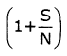; r = C;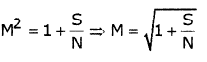QUESTION: 11

The probability of a bit decoded correctly at receiver is `p'. If n bits are sent, what is the probability that one bit is decoded correctly?

Solution:

correct : p; error: 1-p

out of n bits, only one bit is decoded correctly=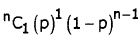QUESTION: 12

A stationary random process x(t) has the following correlation function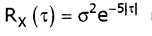is constant) . Find the psd of random signal x(t).

Solution:

PSD of input is expressed as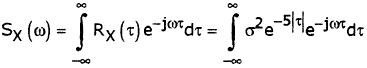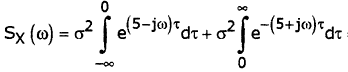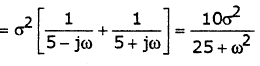QUESTION: 13

If random variable 'x' has Gaussian pdf with mean M, then y=2x has PDF andmean respectively

Solution: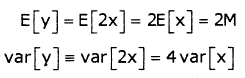so y has pdf N(2m,402) which is Gaussian

QUESTION: 14

If x has P.S.D Sxx (f), then y has P.S.D of ( Assume H(f) is the Fourier Transform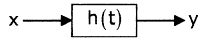Solution: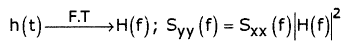QUESTION: 15

A Gaussian random variable 'x' has mean 1 and variance√2. If y = x2, then the mean of y is

Solution: